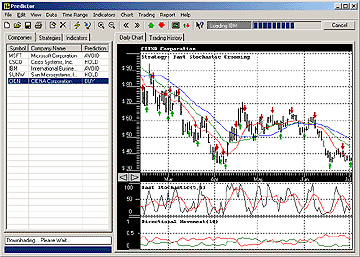# Fast Stochastic Oscillator Cross - Rising

This strategy is based on crossings in Fast Stochastic Oscillator. Typically a buy is generated when a %K (black) line is crossing a %D (red)  line in an upward direction. When %K is crossing %D in a downward direction it is a sell signal.

This strategy is generating a buy signal on an upward crossing of %D by %K and only if that crossing occurs below a certain level a. A sell signal is generated when %D is crossed by %K in a downward direction above a certain level b. Typically a = 20 and b = 80.

### Formula

IF STO%K < a AND STO%D < a
AND STO%D >= STO%K
THEN GO LONG
ELSE
IF STO%K > b AND STO%D > b
AND STO%D <= STO%K
THEN GO SHORT
where STO%K is %K fast stochastic oscillator;
where STO%D is %D fast stochastic oscillator;
and a < b; e.g. a = 20 and b = 80

NVDA BBY KLAC XLNX KO BRCM SLB SEBL BAC FBF

< Back to Stock Predictor SiteOrder >